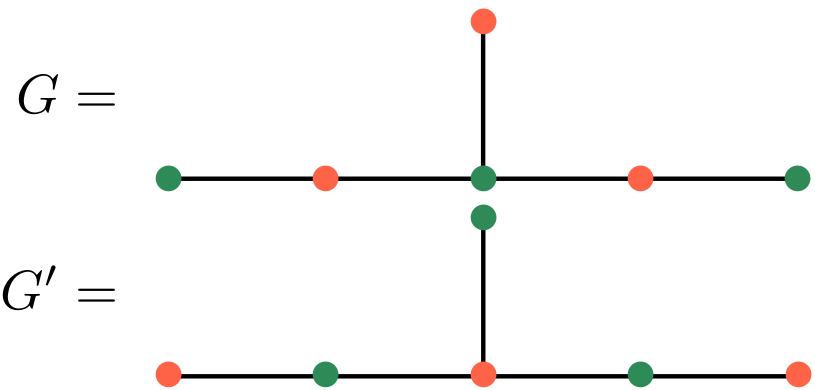## Abstract

In this paper we complete the $$\mathrm{ADE}$$-like classification of simple transitive 2-representations of Soergel bimodules in finite dihedral type, under the assumption of gradeability. In particular, we use bipartite graphs and zigzag algebras of $$\mathrm{ADE}$$ type to give an explicit construction of a graded (non-strict) version of all these 2-representations.
Moreover, we give simple combinatorial criteria for when two such 2-representations are equivalent and for when their Grothendieck groups give rise to isomorphic representations.
Finally, our construction also gives a large class of simple transitive 2-representations in infinite dihedral type for general bipartite graphs.

## A few extra words

An essential problem in classical representation theory is the classification of the simple representations of any given algebra, i.e. the parametrization of their isomorphism classes and the explicit construction of a representative of each class.
Basically the same question arises in 2-representation theory, where the actions of algebras on vector spaces are replaced by functorial actions of 2-categories on certain 2-categories. Examples are 2-representations of the 2-categories which categorify representations of quantum groups, due to (Chuang-)Rouquier and Khovanov-Lauda, and 2-representations of the 2-category of Soergel bimodules, which categorify representations of Hecke algebras.
An appropriate categorical analogue of the simple representations of finite-dimensional algebras are the so-called simple transitive 2-representations (of finitary 2-categories). The problem of their classification is very hard in general and not well understood.
In this paper, we construct all (graded) simple transitive 2-representations (of the small quotient) of the Soergel bimodules of dihedral type (including the infinite dihedral type) by using the so-called two-color Soergel calculus.
Our construction is completely explicit and emphasizes the usage of bipartite graphs which we use to cook up algebras and the 2-categories of their projective endofunctors, on which the two-color Soergel calculus acts.
The main results are then that we can show that two simple transitive 2-representations are equivalent if and only if the corresponding bipartite graphs are isomorphic (as bipartite graphs), and all such 2-representations arise in this way.
Finally, we also determine when simple transitive 2-representations decategorify to isomorphic representations of the corresponding Hecke algebra, using a purely graph-theoretic property.
Hereby we obtain examples of inequivalent simple transitive 2-representations of the same 2-category which decategorify to isomorphic representations, e.g.:The to the bipartite graphs $$G$$ and $$G^{\prime}$$ associated 2-representations are inequivalent, but categorify the same module of the associated dihedral group.